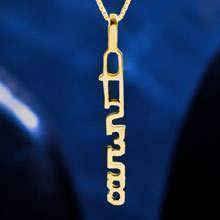# フィボナッチ・シーケンス・ペンダント　ゴールド

### すべての自然界に存在するフィボナッチと数学は、あなたの周囲にあるすべての自然との統一と深いつながりを見つける手助けをしてくれます

This Fibonacci sequence pendant was designed by Gary Meisner who won David's jewelry design contest for April-May 2007. And here is how Gary describes it: "The first numbers of the Fibonacci Series (0, 1, 1, 2, 3, 5, and 8) joined to form a solid chain, with the 0 serving as the eye of the pendant."

The Fibonacci numbers are a sequence in which every number is the sum of the previous two. The Fibonacci numbers were originally implemented by Leonardo Fibonacci to portray the population growth of rabbits.

The Fibonacci sequence appears in many interesting phenomena, for example:

• Determine tuning at music instruments.
• In visual art.
• We encounter it in many natural and biological processes, i.e. it shows up in the spirals of seashells, the curve of waves, the growth of tree branches and many more natural phenomemnon.

It's most interesting relation is to the Golden mean number: Phi. If you divide each number in the series with the number preceding it, the products get closer and closer to Phi (1.618…):
1/1=1
2/1=2
3/2=1.5
5/3=1.66666
8/5=1.6
13/8=1.625
21/13=1.615384
And so on…

The Fibonacci sequence is the mathematical basis for so many occurrences that exist in our world.